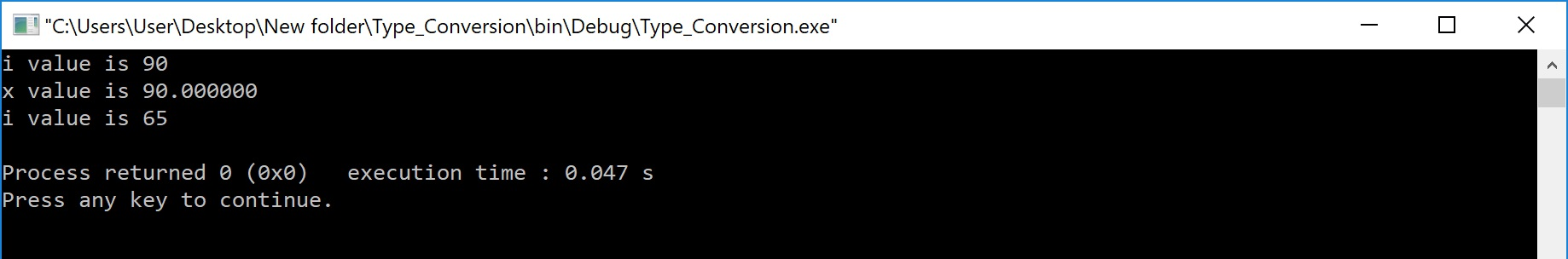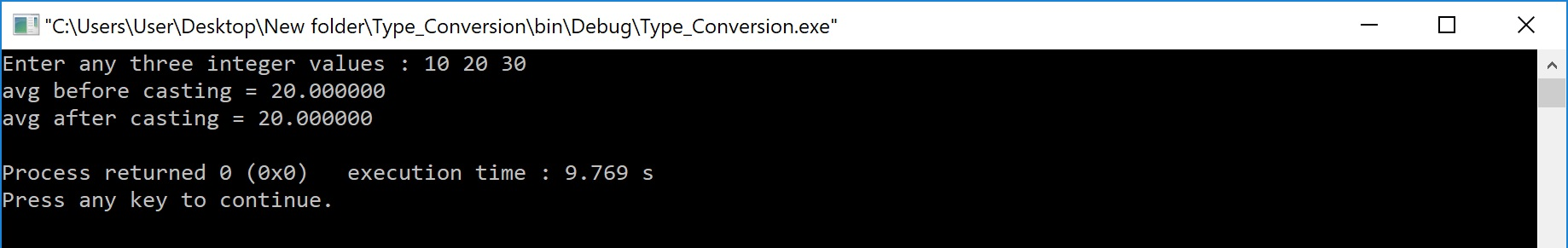The perfect place for easy learning...

×

# Type Casting and Conversion in C

In a programming language, the expression contains data values of the same datatype or different data types. When the expression contains similar datatype values then it is evaluated without any problem. But if the expression contains two or more different datatype values then they must be converted to the single datatype of destination datatype. Here, the destination is the location where the final result of that expression is stored. For example, the multiplication of an integer data value with the float data value and storing the result into a float variable. In this case, the integer value must be converted to float value so that the final result is a float datatype value.
In a c programming language, the data conversion is performed in two different methods as follows...

1. Type Conversion
2. Type Casting

# Type Conversion

The type conversion is the process of converting a data value from one data type to another data type automatically by the compiler. Sometimes type conversion is also called implicit type conversion. The implicit type conversion is automatically performed by the compiler.
For example, in c programming language, when we assign an integer value to a float variable the integer value automatically gets converted to float value by adding decimal value 0. And when a float value is assigned to an integer variable the float value automatically gets converted to an integer value by removing the decimal value. To understand more about type conversion observe the following...

int i = 10 ;
float x = 15.5 ;
char ch = 'A' ;

i = x ; =======> x value 15.5 is converted as 15 and assigned to variable i

x = i ; =======> Here i value 10 is converted as 10.000000 and assigned to variable x

i = ch ; =======> Here the ASCII value of A (65) is assigned to i

# Example Program

``````#include<stdio.h>
#include<conio.h>

void main(){
int i = 95 ;
float x = 90.99 ;
char ch = 'A' ;

i = x ;
printf("i value is %d\n",i);
x = i ;
printf("x value is %f\n",x);
i = ch ;
printf("i value is %d\n",i);

}```
```

Output:In the above program, we assign i = x, i.e., float variable value is assigned to the integer variable. Here, the compiler automatically converts the float value (90.99) into integer value (90) by removing the decimal part of the float value (90.99) and then it is assigned to variable i. Similarly, when we assign x = i, the integer value (90) gets converted to float value (90.000000) by adding zero as the decimal part.

# Typecasting

Typecasting is also called an explicit type conversion. Compiler converts data from one data type to another data type implicitly. When compiler converts implicitly, there may be a data loss. In such a case, we convert the data from one data type to another data type using explicit type conversion. To perform this we use the unary cast operator. To convert data from one type to another type we specify the target data type in parenthesis as a prefix to the data value that has to be converted. The general syntax of typecasting is as follows.

(TargetDatatype) DataValue

# Example

int totalMarks = 450, maxMarks = 600 ;
float average ;

average = (float) totalMarks / maxMarks * 100 ;

In the above example code, both totalMarks and maxMarks are integer data values. When we perform totalMarks / maxMarks the result is a float value, but the destination (average) datatype is a float. So we use type casting to convert totalMarks and maxMarks into float data type.

# Example Program

``````#include<stdio.h>
#include<conio.h>

void main(){
int a, b, c ;
float avg ;
cout << "Enter any three integer values : ";
cin >> a >> b >> c;

avg = (a + b + c) / 3 ;
cout << "avg before casting = " << avg << endl;

avg = (float)(a + b + c) / 3 ;
cout << "avg after casting = " << avg << endl;

return 0;
}```
```

Output: